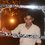# Basics: Applications of derivatives

I've started studying the applications of derivatives and I'm not so fluent about the concepts. Could you please help me? Here are the some problems I'm struggling with,

(1) If $$f\left( x \right) =2{ e }^{ x }-a{ e }^{ -x }+\left( 2a+1 \right) x-3$$ monotonically increases$$\forall x\epsilon R$$ ,then find the range of values of $$a$$

(2) If $f\left( x \right) ={ e }^{ 2x }-a{ e }^{ x }+1$, prove that $f\left( x \right)$ cannot be monotonically decreases for $\forall x\epsilon R$ for any value of $a$.

(3) The values of $a$ for which $f\left( x \right) =\left( a+2 \right) { x }^{ 3 }-a{ x }^{ 2 }=9ax-1$ monotonically decreasing.

(4) Let $f\left( x \right) =\ \begin{Bmatrix} { x }^{ 2 }+x\quad \quad ;-1\le x<0 \\ \lambda ;x=0 \\ \log _{ 1/2 }{ ( x+\frac { 1 }{ 2 } ) ;0 . Discuss global maxima, minima for $\lambda=0$ and $\lambda =1$. For what values of $=0$ does $f\left( x \right)$ has global maxima?Note by Anandhu Raj
5 years, 6 months ago

This discussion board is a place to discuss our Daily Challenges and the math and science related to those challenges. Explanations are more than just a solution — they should explain the steps and thinking strategies that you used to obtain the solution. Comments should further the discussion of math and science.

When posting on Brilliant:

• Use the emojis to react to an explanation, whether you're congratulating a job well done , or just really confused .
• Ask specific questions about the challenge or the steps in somebody's explanation. Well-posed questions can add a lot to the discussion, but posting "I don't understand!" doesn't help anyone.
• Try to contribute something new to the discussion, whether it is an extension, generalization or other idea related to the challenge.
• Stay on topic — we're all here to learn more about math and science, not to hear about your favorite get-rich-quick scheme or current world events.

MarkdownAppears as
*italics* or _italics_ italics
**bold** or __bold__ bold
- bulleted- list
• bulleted
• list
1. numbered2. list
1. numbered
2. list
Note: you must add a full line of space before and after lists for them to show up correctly
paragraph 1paragraph 2

paragraph 1

paragraph 2

[example link](https://brilliant.org)example link
> This is a quote
This is a quote
    # I indented these lines
# 4 spaces, and now they show
# up as a code block.

print "hello world"
# I indented these lines
# 4 spaces, and now they show
# up as a code block.

print "hello world"
MathAppears as
Remember to wrap math in $$ ... $$ or $ ... $ to ensure proper formatting.
2 \times 3 $2 \times 3$
2^{34} $2^{34}$
a_{i-1} $a_{i-1}$
\frac{2}{3} $\frac{2}{3}$
\sqrt{2} $\sqrt{2}$
\sum_{i=1}^3 $\sum_{i=1}^3$
\sin \theta $\sin \theta$
\boxed{123} $\boxed{123}$

## Comments

Sort by:

Top Newest

(1) $f(x) = 2e^x - ae^{-x} + (2a + 1)x - 3$
A function is said to be monotonically increasing if $f'(x)$ > 0 for all real x .
$\Rightarrow f'(x) = 2e^x + ae^x + (2a+1) > 0$
$\Rightarrow a < \dfrac{2e^x +1}{-e^x -2}$
$\Rightarrow -\dfrac{1}{2} < a < \infty \quad \quad \quad (\text{From the graph})$

Similarly, you can try other parts.

- 5 years, 6 months ago

Log in to reply

×

Problem Loading...

Note Loading...

Set Loading...